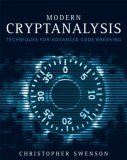computer science, math, programming and other stuff
a blog by Christopher Swenson

# Modern Cryptanalysis## Errata

I (and others) have noted several typos and other small errors in Modern Cryptanalysis. I will post them here as I find out about them.

Feel free to email me more at chris@caswenson.com.

• p. 6, third paragraph under 1.2.3 Klingon, where the Klingon should have the apostrophe after the "y", since the apostrophe is a separate character.
• p. 6, Table 1-2, it contains both a capital "i" (I) and a lowercase "L" (l), which happen look nearly identical in that font.
• p. 14, paragraph 5, first sentence should read "This gives us a the following formula to calculate the theoretical minimum." (instead of maximum).
• p. 16, in the paragraph starting with "Freidrich Kasiski", 4th sentence should state "n + 1" times instead of "n" times, in order to guarantee a repeat.
• p. 35, same error in the first paragraph (I must have been on a roll), where you need 367 people to guarantee a collision (because of leap years).
• p. 43, second paragraph, g and m must be relatively prime for us to invoke Euler's totient theorem.
• p. 45, 4th paragraph, "(Z + 1)" should be ", for example, (Z, +)".
• p. 46, I forgot to note that 6 is its own inverse mod 7.
• p. 46, directly under that, the definition of GF(n) should start with "({0, 1, ..., n - 1}", (second curly brace got flipped).
• p. 47, first paragraph, the sentence "This way, n, ..." should be "This way, n_0...".
• p. 47, third formula, the "i + 2" should be "i + 1" as a subscrpt of "n".
• p. 47, second-to-last paragraph, it should read "1 = a x a^{-1} - kp".
• p. 49, I cheated a little and didn't use the Chinese Remainder Theorem, so I have a few more assumptions in my "proof", in that M and n need to be relatively prime. My bad.
• p. 52, I accidentally reversed the computations for Alice and Bob in the paragraph immediately after the algorithm, since Alice has can compute (g^b)^a, and Bob can compute (g^a)^b, not vice versa.
• p. 58, steps 5 and 6, "a" should be "u". Also, the algorithm is incorrectly stated. See reference  for a better version, and I will correct it here soon.
• p. 70, the logarithms are natural logarithms.
• p. 74, the code should set b = 2, rather than 3, under "def f(x, n)".
• p. 75, Pollard's p-1 algorithm, step 3, it should be "Set g to be the GCD of a and b - 1".
• p. 100, the Skipjack description is incorrect: the diagram is correct though. (Some of the w's got mixed up in the text.)

Thanks to Chris Miller and Jack Schlesinger for helping me locate these.